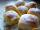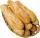Denominator

Calculate the missing denominator x:Result

x =  4

Solution:Leave us a comment of example and its solution (i.e. if it is still somewhat unclear...):Be the first to comment!To solve this verbal math problem are needed these knowledge from mathematics:

Need help calculate sum, simplify or multiply fractions? Try our fraction calculator. Do you have a linear equation or system of equations and looking for its solution? Or do you have quadratic equation?

Next similar examples:

1. Jam cakesMom baked a third of plum jam cakes, one third cheesecakes and 18 poppy. How many cakes she had bake?
2. Equation 20In given equation: 8/9-4/5=2/9+x, find x
3. Cakes1/3 poppy cake, 1/3 apple, 15 pieces of cheese. How many are totally cakes?Mom bake cookies. Rolo took 2/9 of all cookies, Michal 3/9. How many cookies ate Rolo if Michal had 9.
5. If-then equationIf 5x - 17 = -x + 7, then x =
6. Forest nurseryIn the forest nursery after winter, they found that 1/10 stems died out of them. For them, they land 193 new spruces. How many spruces are in the forest nursery?
7. Eq1Solve equation: 4(a-3)=3(2a-5)
8. In fractionsAn ant climbs 2/5 of the pole on the first hour and climbs 1/4 of the pole on the next hour. What part of the pole does the ant climb in two hours?
9. RollsMom bought 13 rolls. Dad ate 3.5 rolls. How many rolls left when Peter yet put two at dinner?
10. Fraction to decimalWrite the fraction 3/22 as a decimal.
11. CakesOn the bowl were a few cakes. Jane ate one-third of them, Dana ate a quarter of those cakes that remained. a) What part (of the original number of cakes) Dana ate? b) At least how many cakes could be (initially) on thebowl?
12. ZdeněkZdeněk picked up 15 l of water from a 100-liter full-water barrel. Write a fraction of what part of Zdeněk's water he picked.
13. Reduce to lowest termsReduce to lowest terms : 32/124
14. Mixed2improperWrite the mixed number as an improper fraction. 166 2/3
15. Lengths of the poolMiguel swam 6 lengths of the pool. Mat swam 3 times as far as Miguel. Lionel swam 1/3 as far as Miguel. How many lengths did mat swim?
16. Fraction and a decimalWrite as a fraction and a decimal. One and two plus three and five hundredthsAdded together and write as decimal number: LXVII + MLXIV